## Concept of Stress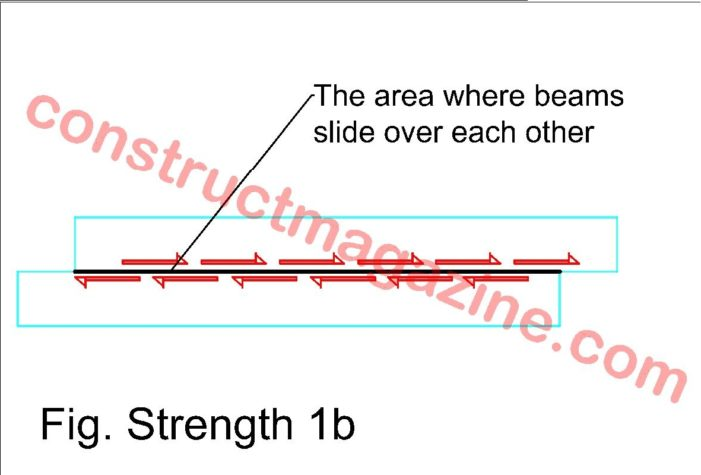Many things in civil engineering are expressed in terms of stress, rather than forces (loads). The stress (pressure) is found by dividing the force (load) by area. So, Stress = Force / Area So it has units of Newtons per milimeter square, N/mm2 (Mega Pascal – MPa), or pounds per inch square,  lbs/inch2 (psi) for […]

## Strength / Mechanics of Materials

In previous section, we dealt with external forces acting on bodies, but we didn’t care about the actual material itself. We thought about ideally rigid materials. We did not consider how strong were our materials, what happens to materials, when forces act on them, how much they deform or and at what point they break. […]

## Determinate and Indeterminate Structures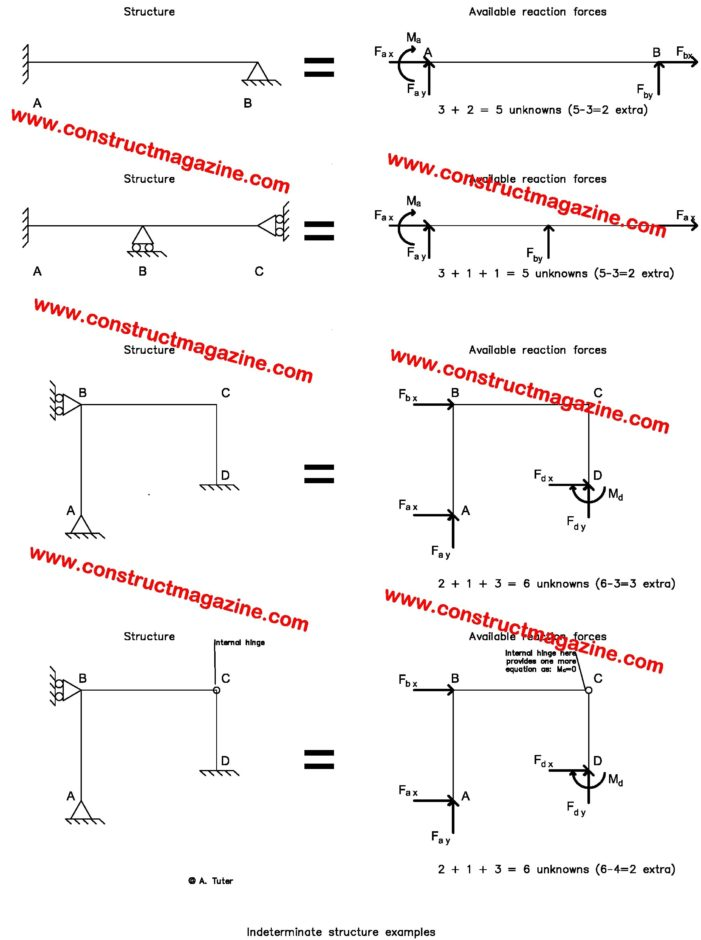One more post and we will finish the statics subject of our post series… The beam example we have just given in previous post, was a simple beam. In that problem, we were easily able to solve all reactions, just by applying three equations, which were, Total Fx = 0 Total Fy = 0 Total […]

## Axial Force, Shear Force, Bending Moment Diagrams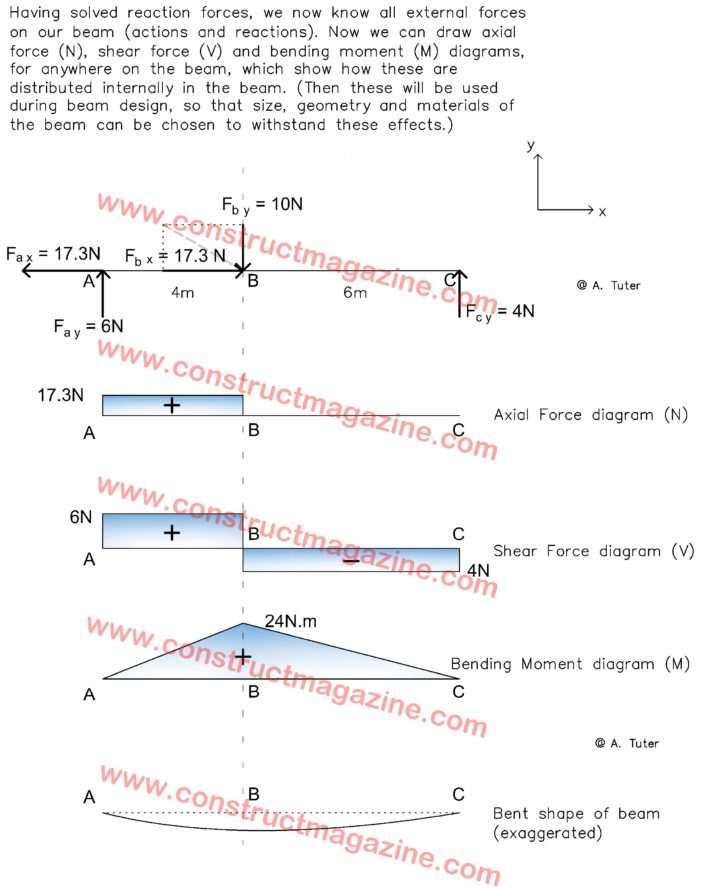So far you have learned very important and fundamental concepts in statics. Now lets go one step further and introduce another fundamental subject. The force and moment diagrams. In the example in the previous post of this series, we had a beam, which was shown with its supports and an external force acting on it, […]

## Example for solving reactions in statics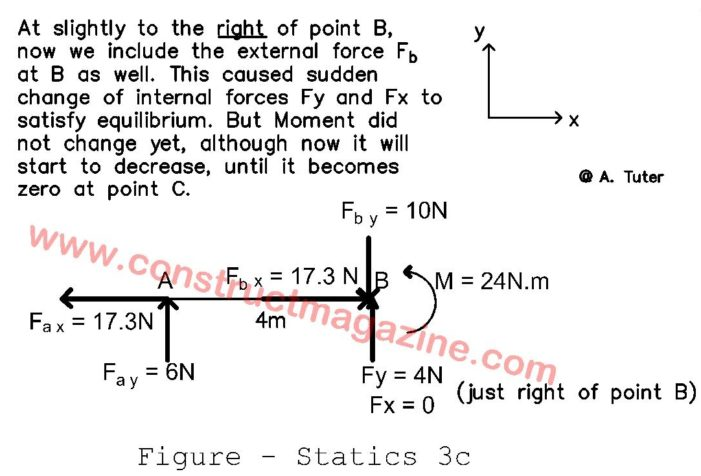Example for solving reactions: Now let’s do a simple example, to better digest everything we have learned so far… Let’s calculate reaction forces on a beam, based on given external forces. Assuming you are not an engineer, if you do not understand this example, do not spend too much time on it, although it is […]

## Types of supports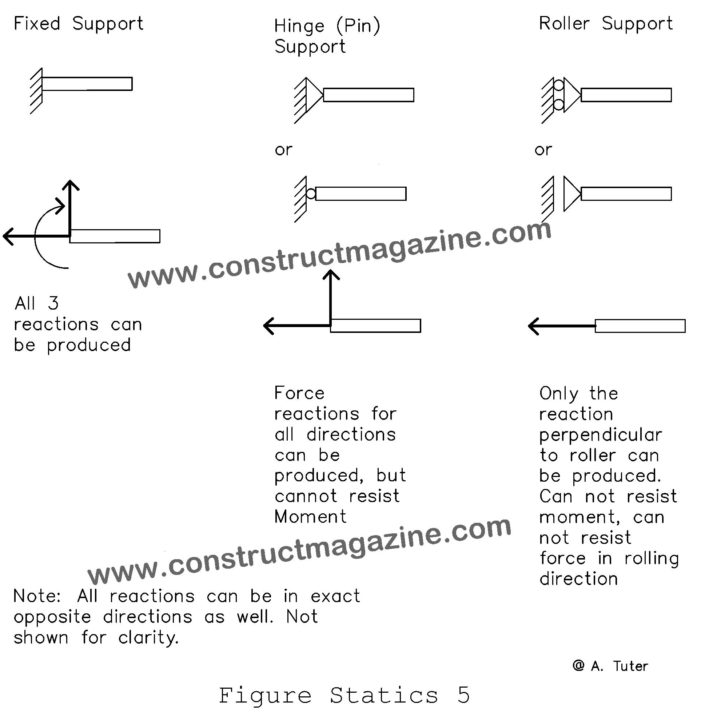Although we described the concept already, lets summarize here the three types of supports you see in structures and how they are shown: Note that if in real life the beam had supports like that, in fixed support case, it would not move. In hinge supported case, it would only rotate around hinge, as there […]

## Equlibrium Concept, Action / Reaction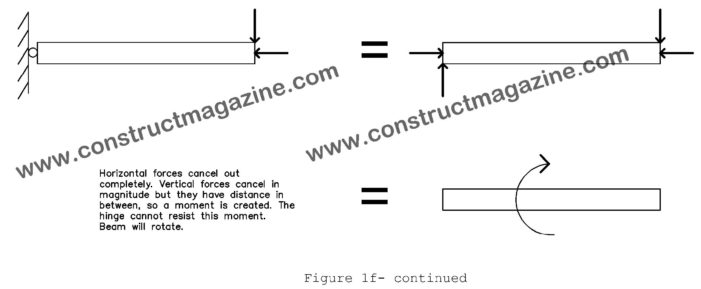As Sir Isaac Newton discovered centuries ago, a force, (if unbalanced by an equal reaction acting on the opposite direction) will create an acceleration on the body, as in F=ma, where F is the force in newtons, m is the mass in kg and a is acceleration in m/s2. Same is true for turning effects. […]

## Forces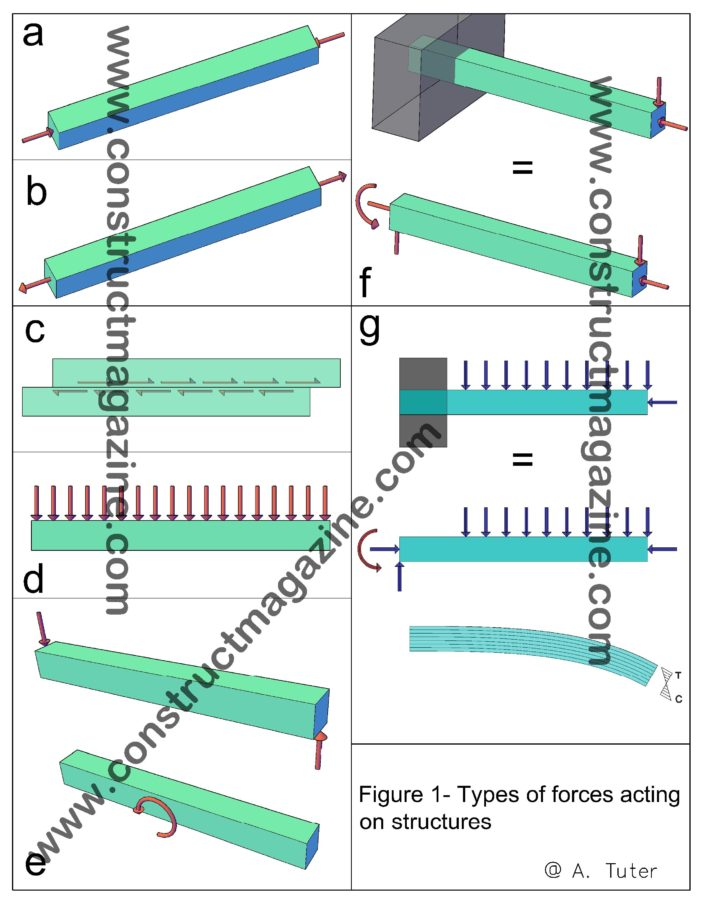Forces can be as: Compressive Tensile Shear All loads acting on structures are a combination of these forces. In addition, a force can also create a turning affect around a point, which can be of two types, as: Bending Moment (or simply “Moment” because it is very frequent) Torsional Moment (os simply “Torsion”, because it […]

## Structural Basics: Forces and StressesIntroduction As we mentioned before, civil engineering is divided into five main branches. Now we will start to introduce the structural branch, and show the basics of probably the most two fundamental concepts in civil engineering, which are used in all branches of civil engineering as well, and not only structural.  These are forces and […]